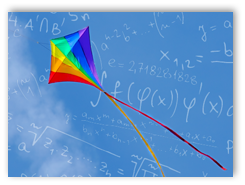Flying kites is one of America’s favorite past times. We love buying, or making, that special kite. We wait and wait for a windy day. We grab our kite and head to the park. We can feel the excitement as we start to unwind the string. We check the direction of the wind and hold the kite up and start running. As we run the kite starts to fly higher and higher. We turn around and our hearts are filled with joy as we see our kite flying high.

However, the perfect conditions for flying kites is not always present, especially in the state of Colorado. We have many beautiful summer days – with no wind. We have many winter days that are windy, but our local park may have three feet of snow. How, then, do we nurture our inner kite-lover?

## An alternative to the real thing

When we cannot fly a real kite, we can resort to mathematics, where we can model almost everything. A mathematical model is basically an equation, or set of equations, that represents a real life scenario. A simple mathematical model for how much we have to pay at the gas pump, is Y = \$3.79X, where Y is the amount we pay, and X is the number of gallons. In algebra, the notation for multiplication is to write a number, \$3.79, next to the variable (the letter), X. So, if you buy 2 gallons of gas, you will pay \$3.79 times 2, which is \$7.58.

## Using mathematics to model a kite in flight

In order to model a kite in flight, we would need several quantities, such as, forces on the kite, including tension in the string, force due to drag, force due to lift, force due to gravity, wind velocity, and quantities such as, the density of air, the surface area of the kite, and, the mass of the kite. We can combine these quantities with a little physics to get a set of differential equations that, when we enter certain amounts, such as wind speed, we get back the kite’s location, direction, and speed. Real kites can rise, fall, and oscillate. The mathematical model can give us values that we interpret as rising, falling, or oscillating.

## You can create mathematical models for anything

When I was in college taking math classes, I was fascinated by how we can model so many different things in our world. For example, we can model how fast rumors spread through a school, or how fast a virus spreads. We can model forces on a bridge or a building. We can model how much pollution is in a river and how many fish survive in various levels of pollution. We can even model a romantic relationship. Mathematical models of kites, airplanes, and rockets are some of my favorite models.

## FRCC Math classes to help

If you are interested in learning to create mathematical models, we have several math courses as part of our Mathematics program to help you. You can learn the foundational skills in our algebra classes (MAT 090 Elementary Algebra, MAT 099 Intermediation Algebra, and MAT 121 College Algebra). Trigonometry, MAT 122, is needed to model the kite in flight because of the angles involved. Calculus (MAT 201, 202, and 204) and Physics (PHY 111, 112, 211, 212) are needed for many physical models. More sophisticated mathematical models can be created using Linear Algebra (MAT 255) or Differential Equations (MAT 265).

The next time you would like to fly a kite (or fly a plane, kick a soccer ball, or bungee jump off a bridge), you can do so in the comfort of your own home – by using a mathematical model!